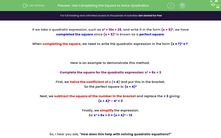# Use Completing the Square to Solve Quadratics

In this worksheet, students will learn how to solve a quadratic equation using the method of completing the square.Key stage:  KS 4

Year:  GCSE

GCSE Subjects:   Maths

GCSE Boards:   Pearson Edexcel, OCR, Eduqas, AQA,

Curriculum topic:   Algebra

Curriculum subtopic:   Solving Equations and Inequalities Algebraic Equations

Difficulty level:#### Worksheet Overview

If we take a quadratic expression, such as x² + 10x + 25, and write it in the form (x + 5)², we have completed the square since (x + 5)² is known as a perfect square.

When completing the square, we need to write the quadratic expression in the form (x ± ?)² ± ?

Here is an example to demonstrate this method.

Complete the square for the quadratic expression: x² + 8x + 3

First, we halve the coefficient of x (+ 4) and put this in the bracket.

So the perfect square is: (x + 4)²

Next, we subtract the square of the number in the bracket and replace the + 3 giving:

(x + 4)² - 4² + 3

Finally, we simplify the expression.

So x² + 8x + 3 = (x + 4)² - 13

So, I hear you ask, "How does this help with solving quadratic equations?"

When trying to solve quadratic equations which will not factorise, completing the square provides us with an alternative method which does not require a calculator, unlike using the quadratic formula.

If the expression above was the equation x² + 8x + 3 = 0, completing the square enables us to solve this using the balancing method as follows:

x² + 8x + 3 = 0

This is the same as: (x + 4)² - 13 = 0

Add 13 to both sides: (x + 4)² = 13

Take the square root of both sides: x + 4 = ±√13

(Don't forget there are two possible square roots: one positive and one negative.)

Subtract 4 from both sides: x = ±√13 - 4

So there are two solutions: x = +√13 - 4 or x = -√13 - 4

These are best left as surds unless you are told to convert them to decimals.

Here is another example to follow through.

Solve the equation x² - 5x - 4 = 0 by completing the square.

Halve the coefficient of x and subtract this value squared: (x - 5/2)² - (5/2)² - 4 = 0

Simplify: (x - 5/2)² - 41/4 = 0

Add 41/4 to both sides: (x - 5/2)² = 41/4

Square root: x - 5/2 = ±√(41/4)

Add 5/2 to both sides: x = 5/2 ±√(41/4) or x = (5 ± √41)/2

So x = (5 + √41)/2 or x = (5 - √41)/2

If there is a coefficient of , then we must divide the whole equation by this first and then continue as before.

By completing the square, solve: 3x² - 12x + 4 = 0

Divide both sides by 3: x² - 4x + 4/3 = 0

Complete the square: (x - 2)² - (-2)² + 4/3 = 0

Simplify: (x - 2)² - 8/3 = 0

Solve: (x - 2)² = 8/3

x - 2 = ±√(8/3)

x = 2 ±√(8/3)

So x = 2 + √(8/3) or x = 2 - √(8/3)

Now it's over to you to solve some quadratic equations using the method of completing the square.

These methods can be long and tricky to remember, so it is a good idea to have a pen and paper handy.

If you write out your method for each question, you can compare your working with what our maths teacher has written.

### What is EdPlace?

We're your National Curriculum aligned online education content provider helping each child succeed in English, maths and science from year 1 to GCSE. With an EdPlace account you’ll be able to track and measure progress, helping each child achieve their best. We build confidence and attainment by personalising each child’s learning at a level that suits them.

Get started